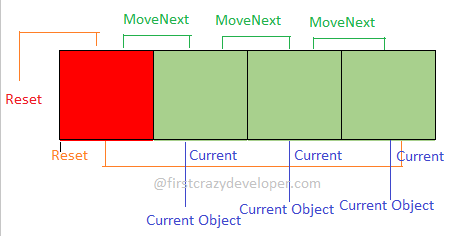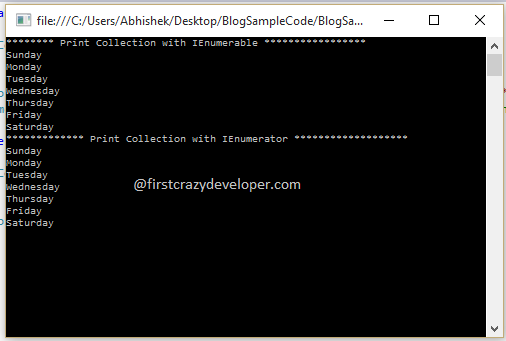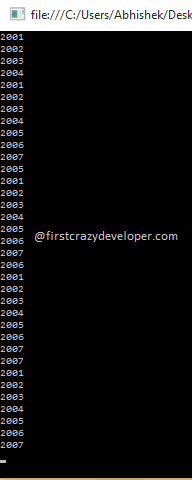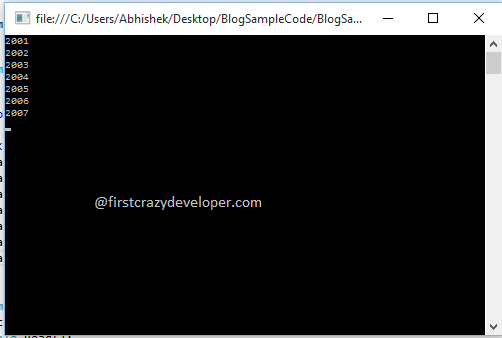© 2021 - First Crazy Developer (Abhishek Kumar)

###IEnumerator v/s IEnumerable in C#

Hi friends, in previous article we discussed about IEnumerable vs IQueryable. Today we move ahead and will start discuss about one more popular comparison -- "IEnumerable vs IEnumerator".

Both are Generic Interface and important with Collection. But most developer confused about their basic differentiate about IEnumerator over IEnumarable Interface.

We use both Interfaces to loop through the Collection. Even internally IEnumerable Interface used IEnumerator.

If we find the defination of IEnumerable Interface we get that it contains an abstract member function called GetEnumerator(). This abstract method helps IEnumerable to iterate over loops. Here we have an important question - "How IEnumerable get this abstract method and where this method defined?"

If we want to find the answer of the above question we need some opeartion over IEnumerator and should look internal structure of this Interface.

Here I have a question to all of you - Have you ever thought of the difference between for loop and foreach? As per my view all C# developer should know this difference.

Presumably, any element in a collection can be retrieved through its index property. But foreach break this myth about iteration.

In the above line we can find the answer of the above question. In for loop we iterate through index but with foreach instead of index we iterate through the help of some abstract method which defined in IEnumerator Interface.

IEnumerator has a method named GetEnumerator(). This method helps to complete iteration without using any index with collection.

Ha ha ha magic in C#.

Look at the following code:

1. public IEnumerator GetEnumerator()
2. {
3. //return IEnumerator of our Custom Type
4. return(IEnumerator)this;
5. }

In the above code we can see the signature of GetEnumerator(). Here GetEnumerator() returns the IEnumerator type of our custom type. Here again we have an important question - "What IEnumerator Interface contains internally?"

IEnumerator Interface contains the following members:

1. void Reset()
2. bool MoveNext()
3. object Current

Now we will discuss about the above members in sequence:

Reset() returns void type and helps us to position the pointer at  the start point.

Look at the following code to understand the Reset() method:

1. public void Reset()
2. {
3. //Get total number of element in a collection
4. length=slist.Count;
5. //Setting the pointer to just before the beginning of collection
6. current=-1;
7. }

In the above code we can see in the first line we set the length of provided Collection and next line set the pointer of the beginning of Collection.

MoveNext() returns Boolean type and help us to check the next element in collections. If there is elements in next position then return true otherwise false.

Look at the following code:

1. public bool MoveNext()
2. {
3. //this will increment the counter variable
4. //and will check whether it is exceeding the actual length of our collection
5. return(++current<length);
6. }

In the above code we can see this method check the pointer position with Collection length and return true or false as per result.

Current returns the element in the collection by using the specified location pointer.

Look at the following code:

1. public object Current
2. {
3. get
4. {
5. //Here "slist" is the collection and "current" is the location pointer
6. return(slist[current]);
7. }
8. }

Now after all of the above discussion we can understand the magic of foreach.

Look at the following image and understand the concepts of IEnumerator.Now I hope we can understand the concepts of IEnumerator and in previous article we already understood the concepts of IEnumerable. We move ahead and will discuss about "when we should prefer IEnumerator over IEnumarable?"

Look at the following code:

1. static void Main(string[] args)
2. {
3. List<string> WeekDays = new List<string>();
11. Console.WriteLine("******** Print Collection with IEnumerable *****************");
12. IEnumerable<string> iEnum = (IEnumerable<string>)WeekDays;
13. foreach(string str in iEnum)
14. {
15. Console.WriteLine(str);
16. }
17. Console.WriteLine("************* Print Collection with IEnumerator *******************");
18. IEnumerator<string> iEnumerat = WeekDays.GetEnumerator();//to convert list into IEnumerator we can invoke the GetEnumerator method
19. while(iEnumerat.MoveNext())
20. {
21. Console.WriteLine(iEnumerat.Current.ToString());
22. }
24. }

In the above code we can see there is string type list named with "WeekDays". Then we added some values with this list. In next line we defined an IEnumerable type variable named "iEnum" then iterate over and print the value. Next we defined variable IEnumerator type named "iEnumerat" and with the help of MoveNext we iterate over collection and help of Current we get the current item and print the value. When we executed the above code we get the following output.

Look at the following image:Here again we have an important question- "What is the difference between them?"

To understand the difference we change the above code. Look at the following code:

1. class Program
2. {
3. static void Main(string[] args)
4. {
5. List<int> myYears = new List<int>();
13. IEnumerable<int> iEnumerat = (IEnumerable<int>)myYears;
14. PrintFirstThreeValues(iEnumerat);
16. }
17. static void PrintFirstThreeValues(IEnumerable<int> Obj)
18. {
19. foreach(int temp in Obj)
20. {
21. Console.WriteLine(temp.ToString());
22. if (temp > 2003)
23. {
24. PrintLastFourValues(Obj);
25. }
26. }
27. }
28. static void PrintLastFourValues(IEnumerable<int> Obj)
29. {
30. foreach (int temp in Obj)
31. {
32. Console.WriteLine(temp.ToString());
33. }
34. }
35. }

In the above code we made some changed. Now we have two more method named "PrintFirstThreeValues" and "PrintLastFourValues". Both method we used the IEnumerable type object as parameter. In "PrintFirstThreeValues” we iterate over Collection and print the value and check that if value is greater than 2003 then called the other method "PrintLastFourValues".

When we executed the above code we get the following output:

Look at the following image:Now move ahead and again we made change in our code just used IEnumerator instead of IEnumerable.

Look at the following code:

1. class Program
2. {
3. static void Main(string[] args)
4. {
5. List<int> myYears = new List<int>();
13. IEnumerator<int> iEnumerat = myYears.GetEnumerator();//to convert list into IEnumerator we can invoke the GetEnumerator method
14. PrintFirstThreeValues(iEnumerat);
16. }
17. static void PrintFirstThreeValues(IEnumerator<int> Obj)
18. {
19. while (Obj.MoveNext())
20. {
21. Console.WriteLine(Obj.Current.ToString());
22. if((int)Obj.Current>2003)
23. {
24. PrintLastFourValues(Obj);
25. }
26. }
27. }
28. static void PrintLastFourValues(IEnumerator<int> Obj)
29. {
30. while (Obj.MoveNext())
31. {
32. Console.WriteLine(Obj.Current.ToString());
33. }
34. }
35. }

In the above code we have same method named "PrintFirstThreeValues" and "PrintLastFourValues".  Here we passed IEnumerator type object instead of IEnumerable as parameter with the both method. In "PrintFirstThreeValues” we iterate over collection and print the value and check if value is greater than 2003 then called the other method "PrintLastFourValues".
Now when we executed the above code we get the following output.

Look at the following image:In the above image we can see the output and we surprised if we compared with both output. As per following output we can say that IEnumerator maintain the state of Collection. We can understand this scenario with the help of the above code. We have two method where in the first method we print the value and check if value is greater than specific value then call the second method. If we go with IEnumerable we can see in the second method collection start from the first point but if use IEnumerator both method sync with Collection.

After the above discussion we get the two point which make differeciate between "IEnumerable vs IEnumerator":

1. Whenever we pass IEnumerator to another function, it knows the current position of item/object.
2. Whenever we pass IEnumerable Collection to another function, it doesn't know the current position of item/object (doesn't know which item it’s executing)

Now I hope we got the confort zone where we can decide better one between "IEnumerable vs IEnumerator" as per our requirements.Home Page 27 September 2015